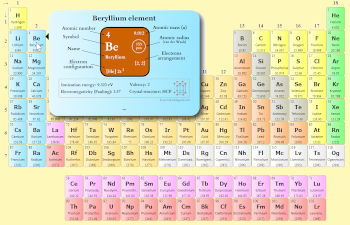# Einsteinium – Periodic Table (Element Information & More)

This is a SUPER easy guide on Einsteinium element.

In fact, the table mentioned below is the perfect information box (Which gives you every single detail about the Einsteinium element in Periodic table.)

So if you want to know anything about the Einsteinium element, then this guide is for you.

Let’s dive right into it!

## Einsteinium Element (Es) InformationSee how this Interactive Periodic Table helps you

• You can effortlessly find every single detail about the elements from this single Interactive Periodic table.
• You will get the detailed information about the periodic table which will convert a newbie into pro.
• You will also get the HD images of the Periodic table (for FREE).

Visit ➢ Periodic table

## Einsteinium in Periodic table

Einsteinium element is in period 7 and in actinides group of the Periodic table. Einsteinium is the f-block element and it belongs to inner transition metals group.

 H He Li Be B C N O F Ne Na Mg Al Si P S Cl Ar K Ca Sc Ti V Cr Mn Fe Co Ni Cu Zn Ga Ge As Se Br Kr Rb Sr Y Zr Nb Mo Tc Ru Rh Pd Ag Cd In Sn Sb Te I Xe Cs Ba La* Hf Ta W Re Os Ir Pt Au Hg Tl Pb Bi Po At Rn Fr Ra Ac** Rf Db Sg Bh Hs Mt Ds Rg Cn Nh Fl Mc Lv Ts Og *Ce Pr Nd Pm Sm Eu Gd Tb Dy Ho Er Tm Yb Lu **Th Pa U Np Pu Am Cm Bk Cf Es Fm Md No Lr

Click on above elements (in Periodic table) to see their information or Visit Interactive Periodic Table (which shows names, symbol, atomic mass, electron configuration, electrons arrangement, etc. of all the elements)

Click on above elements (in Periodic table) to see their information

### Why is Einsteinium in Period 7?

Let me ask you a question.

How many shells does einsteinium have?

It’s 7. Right?

You have already seen the bohr model of einsteinium atom in the above table.

From the Bohr model, it can be found that the number of orbits or shells in einsteinium is 7. Hence, as einsteinium has 7 orbits, it lies in period 7 of the Periodic table.

### Why is Einsteinium in f-block?

Before knowing this reason, first of all I want to ask you a simple question.

How can you determine the blocks-wise position of elements?

The simple answer: The elements will lie in the s, p, d or f block will completely depend upon the subshell in which the last electron will enter.

For example; the electron configuration of einsteinium is [Rn] 5f11 7s2.

So the last electron of einsteinium enters the f-subshell or f-orbital.

Hence, einsteinium is the f-block element.

## 5 Interesting facts about Einsteinium

Interesting facts about einsteinium element are mentioned below.

1. The element was given the name “Einsteinium” to honor the famous physicist Ablert Einstein.
2. Einsteinium was discovered by Albert Ghiorso and his team at Lawrence Berkeley National Laboratory (in 1952).
3. Einsteinium is a radioactive element and it is artificially produced in a nuclear reactor.
4. Total 19 isotopes of einsteinium are known and all these isotopes are radioactive in nature.
5. Out of all the isotopes of einsteinium, the isotope 252Es is the most stable and has a half life of 471 days.

## Properties of Einsteinium

The physical and chemical properties of einsteinium element are mentioned below.

### Physical properties of Einsteinium

Physical properties of einsteinium are mentioned below.

• Einsteinium is a soft metal having a silvery appearance. 
• Einsteinium glows blue in color in the dark, as it releases energy due to radioactive decay.
• The predicted crystal structure of Einsteinium is FCC (face centered cubic).
• The atomic mass of the most stable isotope of einsteinium is 252 u and its density is predicted to be 8.84 g/cm3.
• The calculated melting point of einsteinium is 860 °C.

### Chemical properties of Einsteinium

Chemical properties of einsteinium are mentioned below.

• Einsteinium is a radioactive metal and it shows similar chemicals as that of other actinides.
• Einsteinium shows multiple oxidation states, but the most common oxidation state is +3 and +2. It shows +3 oxidation state in aqueous solutions and it shows +2 oxidation state in solid state.
• The calculated first ionization energy of einsteinium is 6.42 eV.

## Uses of Einsteinium

Einsteinium is generally used for research work in chemistry. But due to its radioactive nature, it has no commercial uses.

## Free Gift for you: Interactive Periodic Table

1).
You can effortlessly find every single detail about the elements from this single Interactive Periodic table.

(For Interactive Periodic table, view on laptop/desktop for better experience. If you are on mobile device, then use a “Desktop site mode” to see interactive periodic table)

2). You will get the detailed information about the periodic table which will convert a newbie into pro.

3). You will also get the HD images of the Periodic table (for FREE).

Checkout Interactive Periodic table and download it’s high resolution image now (It’s FREE)

References:
Chemical data: Wikipedia, Chemspider